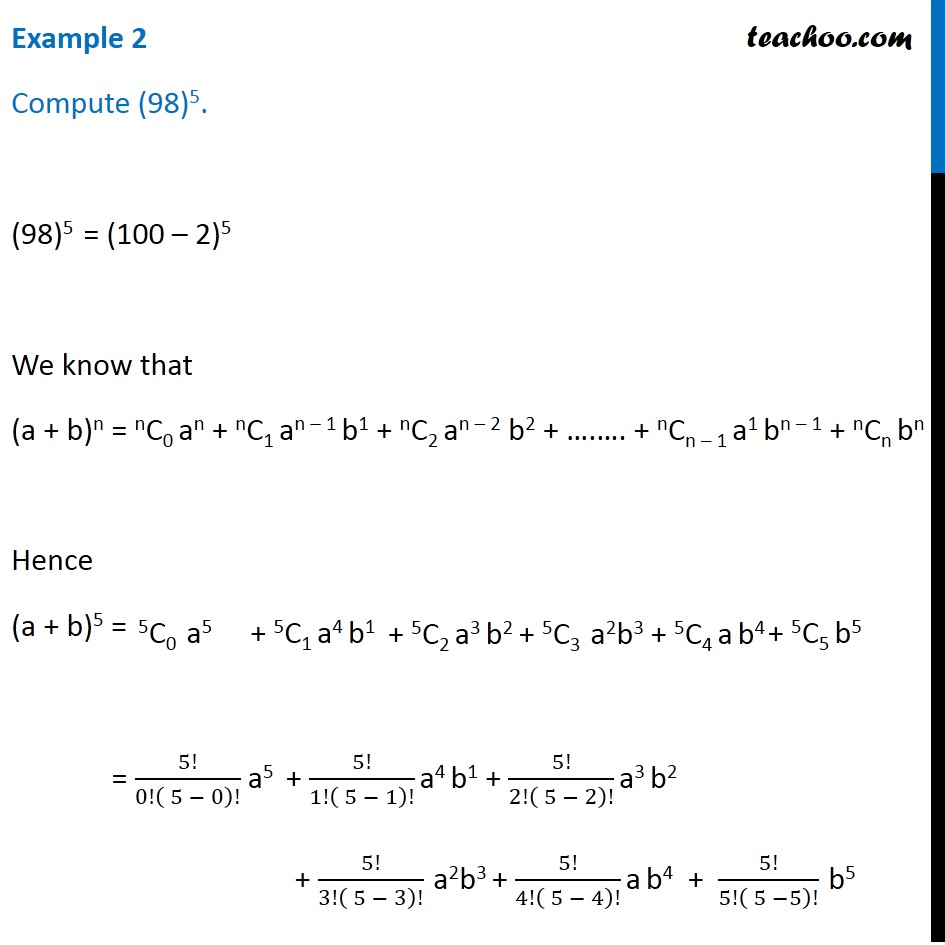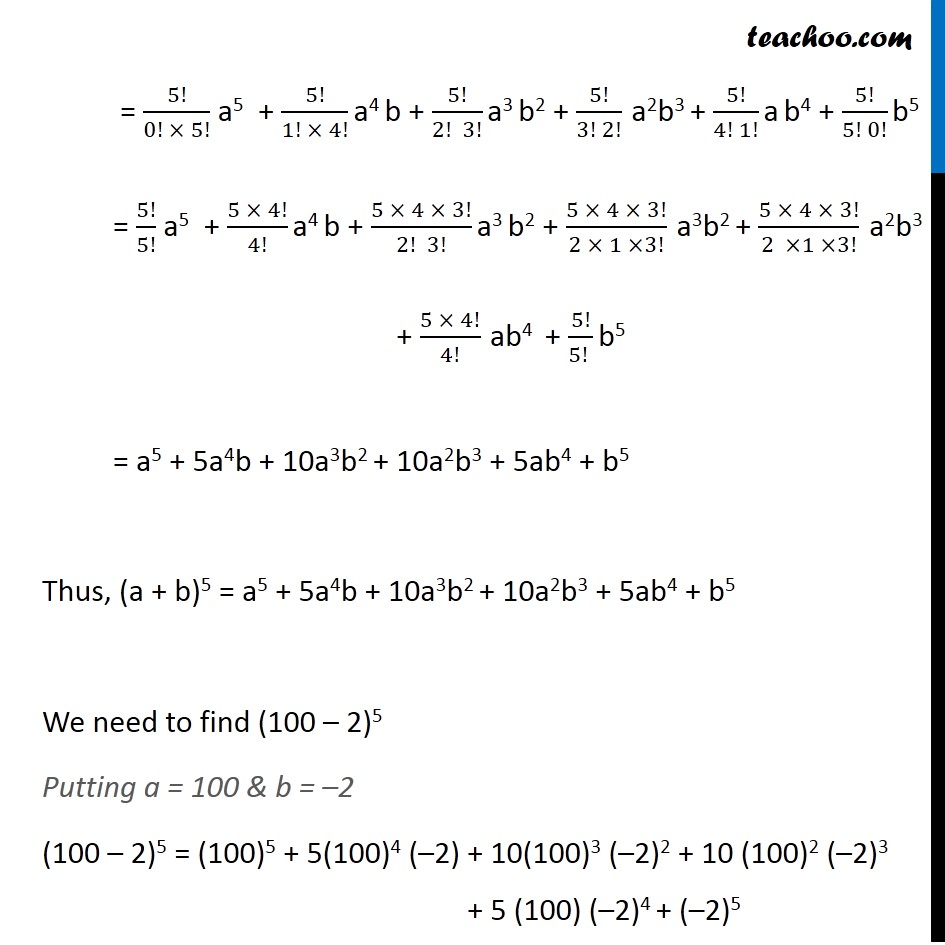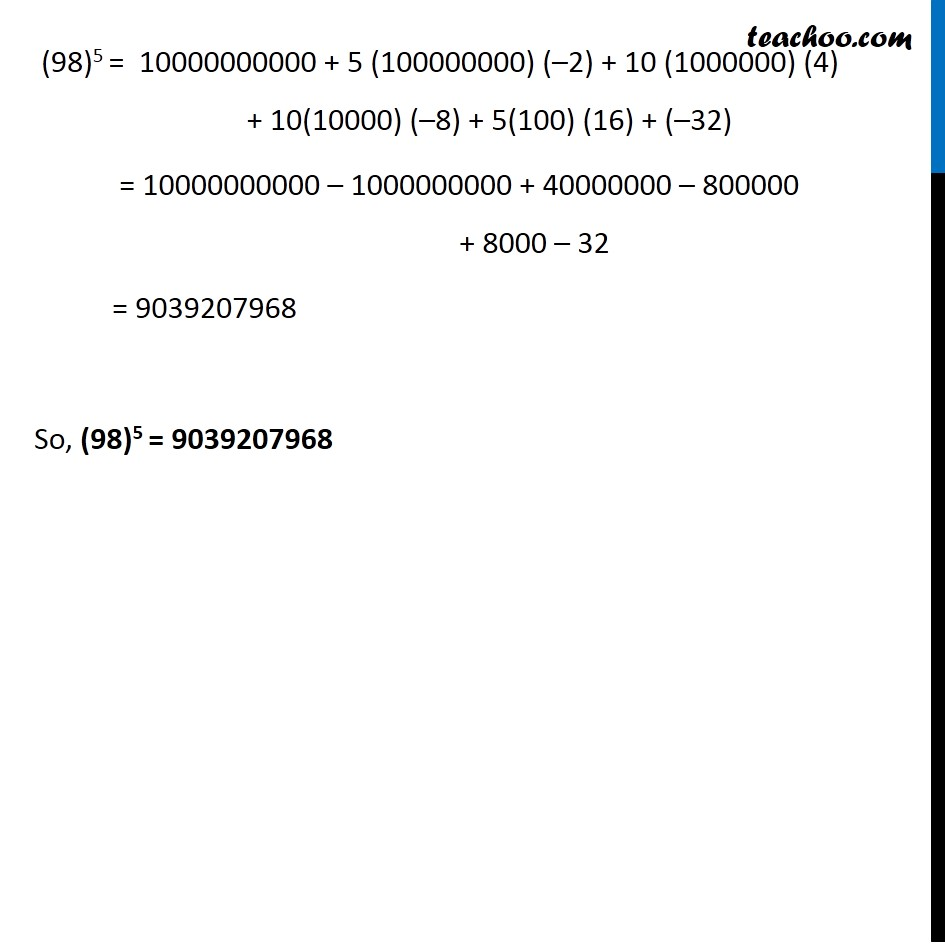Examples

Chapter 7 Class 11 Binomial Theorem
Serial order wiseLearn in your speed, with individual attention - Teachoo Maths 1-on-1 Class

### Transcript

Example 2 Compute (98)5. (98)5 = (100 – 2)5 We know that (a + b)n = nC0 an + nC1 an – 1 b1 + nC2 an – 2 b2 + ….…. + nCn – 1 a1 bn – 1 + nCn bn Hence (a + b)5 = = 5!/0!( 5 − 0)! a5 + 5!/1!( 5 − 1)! a4 b1 + 5!/2!( 5 − 2)! a3 b2 + 5!/3!( 5 − 3)! a2b3 + 5!/4!( 5 − 4)! a b4 + 5!/5!( 5 −5)! b5 = 5!/(0! × 5!) a5 + 5!/(1! × 4!) a4 b + 5!/(2! 3!) a3 b2 + 5!/(3! 2!) a2b3 + 5!/(4! 1!) a b4 + 5!/(5! 0!) b5 = 5!/5! a5 + (5 × 4!)/4! a4 b + (5 × 4 × 3!)/(2! 3!) a3 b2 + (5 × 4 × 3!)/(2 × 1 ×3!) a3b2 + (5 × 4 × 3!)/(2 ×1 ×3!) a2b3 + (5 × 4!)/4! ab4 + 5!/(5! ) b5 = a5 + 5a4b + 10a3b2 + 10a2b3 + 5ab4 + b5 Thus, (a + b)5 = a5 + 5a4b + 10a3b2 + 10a2b3 + 5ab4 + b5 We need to find (100 – 2)5 Putting a = 100 & b = –2 (100 – 2)5 = (100)5 + 5(100)4 (–2) + 10(100)3 (–2)2 + 10 (100)2 (–2)3 + 5 (100) (–2)4 + (–2)5 (98)5 = 10000000000 + 5 (100000000) (–2) + 10 (1000000) (4) + 10(10000) (–8) + 5(100) (16) + (–32) = 10000000000 – 1000000000 + 40000000 – 800000 + 8000 – 32 = 9039207968 So, (98)5 = 9039207968